Flat 25% Off on Your First Order! Use Code : NEWCUST22

# Step-by-Step Guide to Solve Bubble Sort Algorithm in Java

19 Jun 2019 2210 5 minutesBubble Sorting is the primary sorting algorithm that students usually learn during their first semester. It is also known as a sinking sort and is considered as one of the most popular sorting algorithms. Although it is a simple algorithm, still most of the students find it difficult to solve. They run away from it when their professor assigns them Java assignment on bubble sort.

So, in order to make it easier for them, the experts of Global Assignment Help have discussed the step-by-step process to solve the bubble sort in Java.

### How Bubble Sort Algorithm Works?

So, the following list of an array is unsorted. Now, we have to sort the unsorted array in ascending order using the bubble sort algorithm.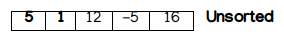#### First Pass:

In the first pass, we will start comparing the elements one by one from the starting. So, let’s start sorting the element using the bubble sort algorithm.

Step 1: Here the algorithm compares the first two elements. If the first element is greater than the second one, then it swaps both the elements.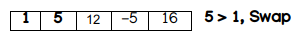Step 2: Now comes the comparison between the next two elements that are 5 and 12. Since 5<12 then="" there="" is="" no="" need="" to="" swap="" the="" elements="" it="" will="" remain="" as="" --12--="">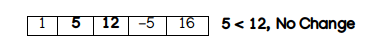Step 3: Now compare the next two elements that is 12 and -5. Since -5 is smaller than 12 so both the elements will be swapped. The list of the array will become:Step 4: Same as the previous step, the next two elements will be compared. Since 12 is smaller than 16 so there will be no change in the list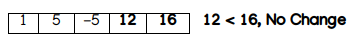#### Second Pass:

OK! So, in the first pass, we have compared all the elements in a line. Now, if we look to the above list of the array, then the list is still unsorted. The reason behind is there is one number with a negative sign. So, we will follow the same process of sorting.

Step 5: Compare the first two elements. Since 1<5, then there will be no change in the list.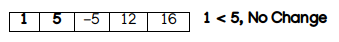Step 6: Now compare the next two elements. Since, 5 > -5, so both the elements will swap. The list will become: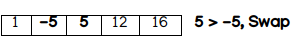Step 7: Same as the previous one, the next two elements will be compared. Since, 5<12, then there will be no change.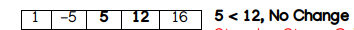Step 8: Now the last two elements will be compared. Since 12<16 it="" will="" remain="" unchanged="" p="">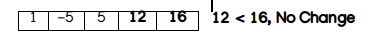#### Third Pass:

Now, this is the last pass to make the list of array fully sorted. So, let’s start from the beginning.

Step 9: Compare the first two elements. Since, 1> -5, then the elements will swap.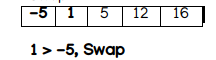Step 10: Similarly, compare the following elements in the same way: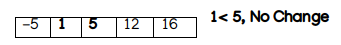Now, we have checked the following list. Since all the elements are sorted, then the list of the array becomes: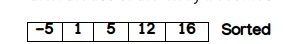Now to implement bubble sort in Java, follow the code given below:

package com.java2novice.algos;

public class MyBubbleSort

{

// logic to sort the elements

public static void bubble_srt(int array[])

{

int n = array.length;

int k;

for (int m = n; m >= 0; m--)

{

for (int i = 0; i < n - 1; i++)

{

k = i + 1;

if (array[i] > array[k])

{

swapNumbers(i, k, array);

}

}

printNumbers(array);

}

}

private static void swapNumbers(int i, int j, int[] array) {

int temp;

temp = array[i];

array[i] = array[j];

array[j] = temp;

}

private static void printNumbers(int[] input) {

for (int i = 0; i < input.length; i++)

{

System.out.print(input[i] + ", ");

}

System.out.println("\n");

}

public static void main(String[] args)

{

int[] input = { 1, 5, -5, 12, 16 };

bubble_srt(input);

}

}

#### Output:

The output will look like this:

1, 5, -5, 12, 16

#### After Sorting:

-5, 1, 5, 12, 16

So, this is all about how you can solve a bubble sort problem in the simplest manner. Going through this blog will surely help you to complete your Java assignment with ease. If still, you face some issues regarding the concepts or anything and are unable to complete your task, then feel free to seek java assignment help from the experts of Global Assignment Help Australia.

Post Comment
Lowest price INR527.84

• 100% Customer Satisfaction
• No Privacy Infringement
• Quick Services
• Subject Experts
• Innovative Documents
FREE Tools

•Paraphrasing Tool

Check your work against paraphrasing & get a free Turnitin report!

Check Paraphrasing
•Plagiarism Checker

Check your work against plagiarism & get a free Turnitin report!

Check Plagiarism
•Reference Generator

Get citations & references in your document in the desired style!

Generate References
•Grammar Checker Tool

•Essay Typer